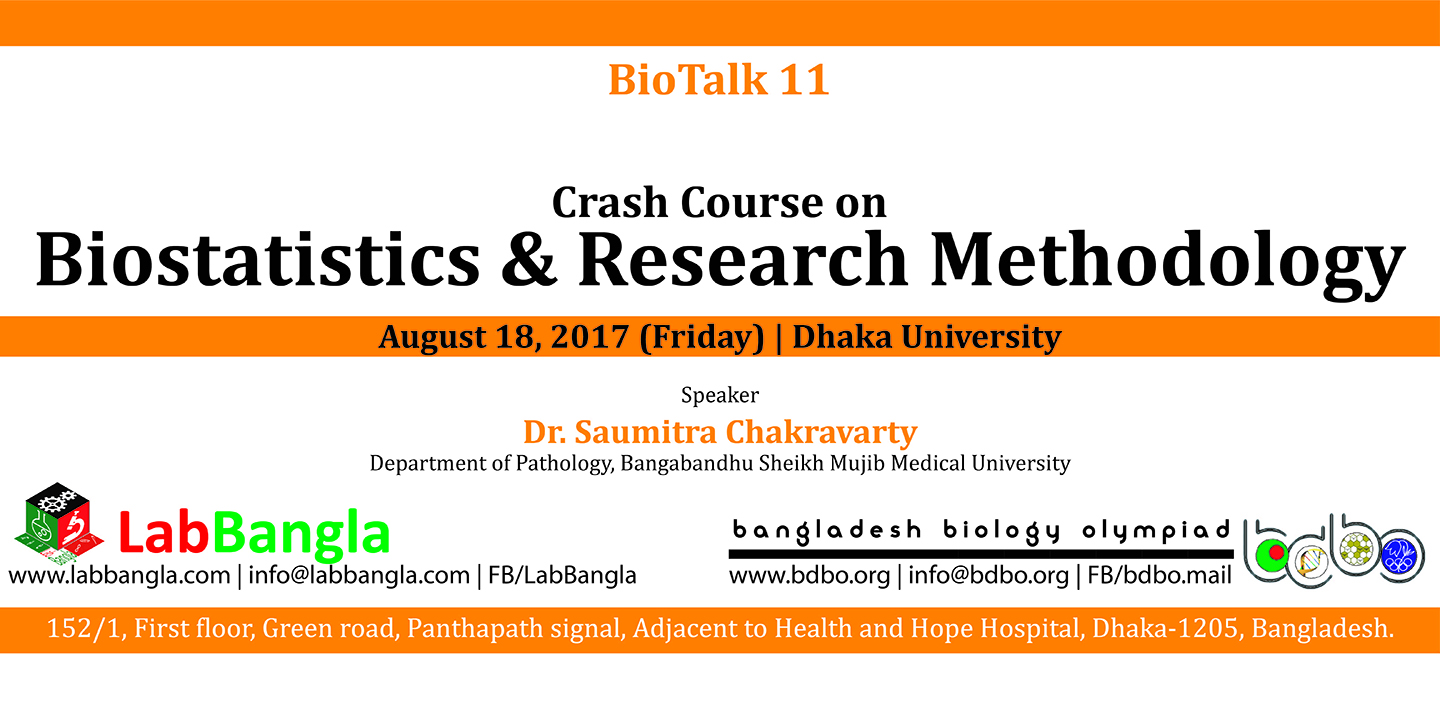11th BioTalk

BioTalk 11Date: 18 August 2017

Duration: 08:30 AM to 04:30 PM

Venue: Dhaka University – Science Cafeteria

Number of registered participants: 102

Participating Universities: Bangladesh Agricultural University, Dhaka University, BRAC University and ASA UniversityModule BST01 [120 minutes]

Why statistics?

Population vs. Sample

Representative sample: What? Why? How?

Variable: Types and importance

Central tendency vs. Dispersion

Mean, median, mode, percentile

Range, variance, deviations

Descriptive statistics vs. Inferential statistics

Module BST02 [120 minutes]

Opinion vs. Fact vs. Hypothesis vs. Theory vs. Law

Hypothesis: Types and formulation

Types of research according to WHO

Probability density function (PDF)

Normal Distribution

Central limit theorem

P-value from normal curve

P-value: What? Why?

Z-transformation

Percentile calculation from Z-value

Confidence interval

Calculation of sample size

Module BST03 [120 minutes]

Z-test for mean

Student’s t-test

Z-test vs. t-test

Chi square test: Two types

Degrees of freedom (DF)

Pearson's Correlation

Association vs. Correlation vs. Causation

Summary